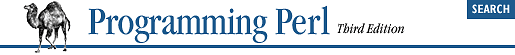home | O'Reilly's CD bookshelfs | FreeBSD | Linux | Cisco | Cisco Exam## 32.33. Math::Complex

```use Math::Complex;

\$z = Math::Complex->make(5, 6);
\$z = cplx(5, 6);         # same thing, but shorter
\$t = 4 - 3*i + \$z;       # do standard complex math
print "\$t\n";            # prints 9+3i

print sqrt(-9), "\n";    # prints 3i```
The Math::Complex module provides complex number objects with overloaded operators. These are numbers with both real and imaginary portions, like those satisfying even-numbered integral roots of negative numbers as shown above. Besides arithmetical operators, many built-in math functions are also overridden by versions that understand complex numbers, including abs, log, sqrt, sin, cos, and atan2. Other functions provided are Re and Im to provide the real and imaginary portions of their complex arguments, plus a full battery of extended trigonometric functions, such as tan, asin, acos, sinh, cosh, and tanh. The module also exports the constant i, which, as you might imagine, holds the value of i; that is, the square root of -1.